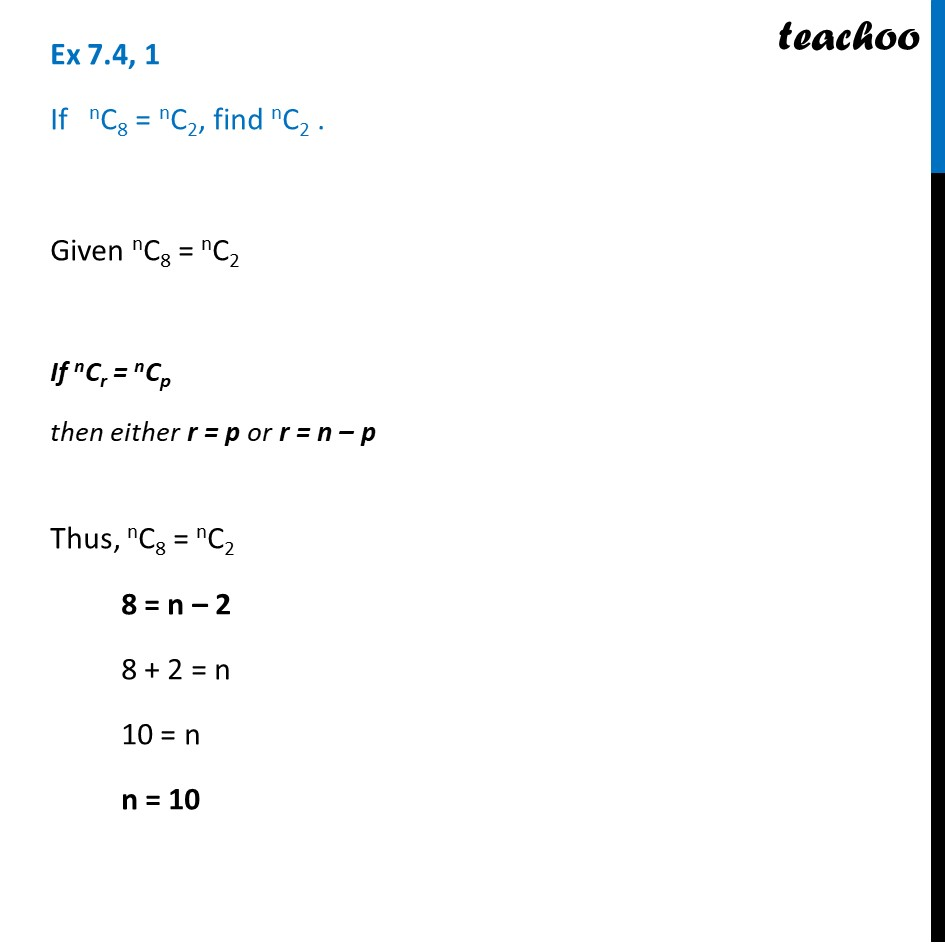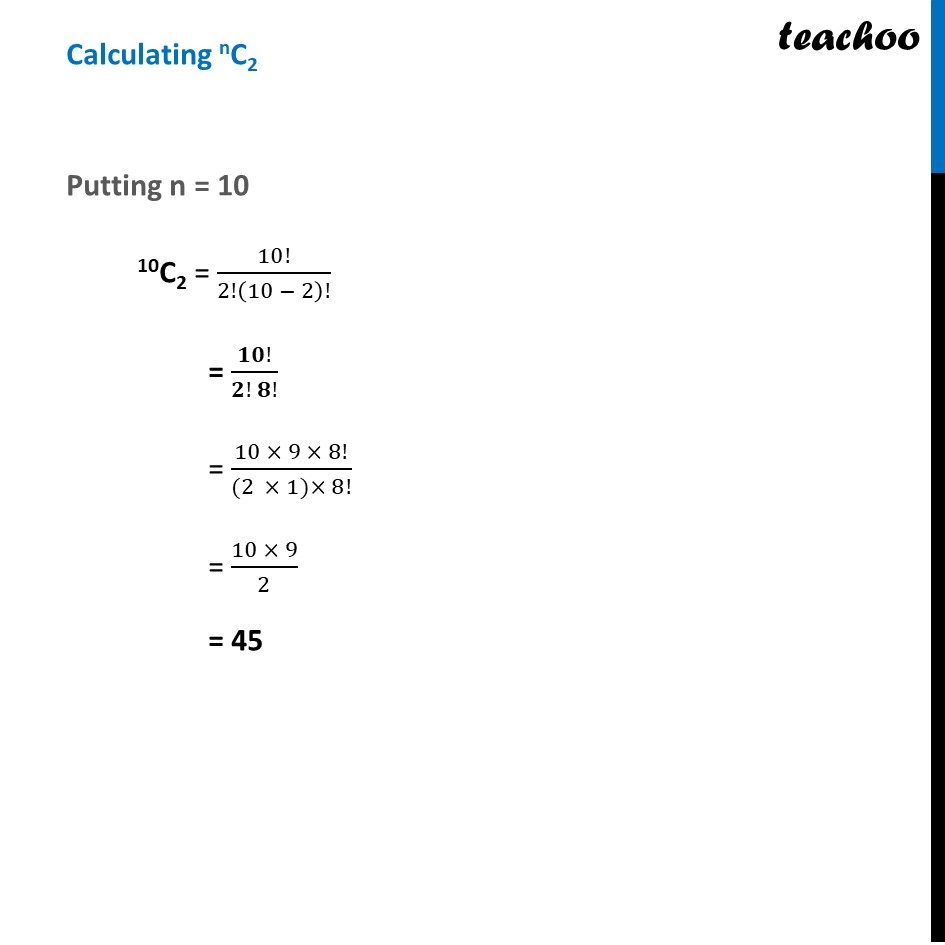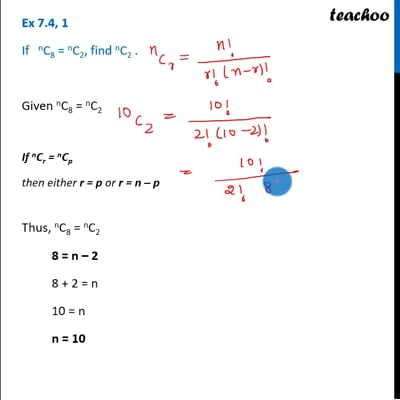Ex 7.4

Chapter 7 Class 11 Permutations and Combinations
Serial order wiseThis video is only available for Teachoo black users

Solve all your doubts with Teachoo Black (new monthly pack available now!)

### Transcript

Ex 7.4, 1 If nC8 = nC2, find nC2 . Given nC8 = nC2 If nCr = nCp then either r = p or r = n – p Thus, nC8 = nC2 8 = n – 2 8 + 2 = n 10 = n n = 10 Calculating nC2 Putting n = 10 10C2 = 10!/2!(10 − 2)! = 𝟏𝟎!/(𝟐! 𝟖!) = (10 × 9 × 8!)/((2 × 1)× 8!) = (10 × 9)/2 = 45